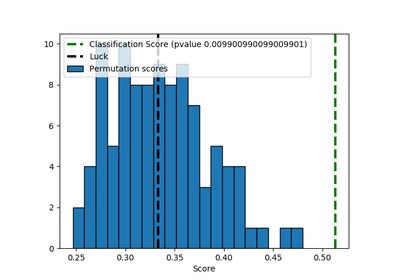# `sklearn.model_selection`.permutation_test_score¶

`sklearn.model_selection.``permutation_test_score`(estimator, X, y, groups=None, cv=None, n_permutations=100, n_jobs=1, random_state=0, verbose=0, scoring=None)[source]

Evaluate the significance of a cross-validated score with permutations

Read more in the User Guide.

Parameters: estimator : estimator object implementing ‘fit’ The object to use to fit the data. X : array-like of shape at least 2D The data to fit. y : array-like The target variable to try to predict in the case of supervised learning. groups : array-like, with shape (n_samples,), optional Labels to constrain permutation within groups, i.e. `y` values are permuted among samples with the same group identifier. When not specified, `y` values are permuted among all samples. When a grouped cross-validator is used, the group labels are also passed on to the `split` method of the cross-validator. The cross-validator uses them for grouping the samples while splitting the dataset into train/test set. scoring : string, callable or None, optional, default: None A single string (see The scoring parameter: defining model evaluation rules) or a callable (see Defining your scoring strategy from metric functions) to evaluate the predictions on the test set. If None the estimator’s default scorer, if available, is used. cv : int, cross-validation generator or an iterable, optional Determines the cross-validation splitting strategy. Possible inputs for cv are: None, to use the default 3-fold cross validation, integer, to specify the number of folds in a (Stratified)KFold, An object to be used as a cross-validation generator. An iterable yielding train, test splits. For integer/None inputs, if the estimator is a classifier and `y` is either binary or multiclass, `StratifiedKFold` is used. In all other cases, `KFold` is used. Refer User Guide for the various cross-validation strategies that can be used here. n_permutations : integer, optional Number of times to permute `y`. n_jobs : integer, optional The number of CPUs to use to do the computation. -1 means ‘all CPUs’. random_state : int, RandomState instance or None, optional (default=0) If int, random_state is the seed used by the random number generator; If RandomState instance, random_state is the random number generator; If None, the random number generator is the RandomState instance used by np.random. verbose : integer, optional The verbosity level. score : float The true score without permuting targets. permutation_scores : array, shape (n_permutations,) The scores obtained for each permutations. pvalue : float The p-value, which approximates the probability that the score would be obtained by chance. This is calculated as: (C + 1) / (n_permutations + 1) Where C is the number of permutations whose score >= the true score. The best possible p-value is 1/(n_permutations + 1), the worst is 1.0.

Notes

This function implements Test 1 in:

Ojala and Garriga. Permutation Tests for Studying Classifier Performance. The Journal of Machine Learning Research (2010) vol. 11

## Examples using `sklearn.model_selection.permutation_test_score`¶Test with permutations the significance of a classification score